# McGraw Hill Math Grade 8 Lesson 21.4 Answer Key Symmetry and Transformations

Practice the questions of McGraw Hill Math Grade 8 Answer Key PDF Lesson 21.4 Symmetry and Transformations to secure good marks & knowledge in the exams.

## McGraw-Hill Math Grade 8 Answer Key Lesson 21.4 Symmetry and Transformations

Exercises

IDENTIFY

For each pair of figures, label as translation, rotation, reflection, or dilation.

Question 1.Translation,

Explanation:
Both the figures are translated of one other because one is a translation of other that is identical duplicate of the other.

Question 2.Rotation,

Explanation:
Fiqures are rotation as a rotation is a type of transformation which is a turn. A figure can be turned clockwise or counterclockwise on the coordinate plane. In both transformations the size and shape of the figure stays exactly the same. A rotation is a transformation that turns the figure in either a clockwise or counterclockwise direction.

Question 3.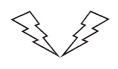Reflection,

Explanation:
Fiqures are reflection as a reflection is a mirror image of the shape. An image will reflect through a line, known as the line of reflection. A figure is said to reflect the other figure, and then every point in a figure is equidistant from each corresponding point in another figure.

Question 4.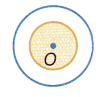Dilation,

Explanation:
Given fiqures are dilation as a dilation is a transformation that enlarges or reduces a figure in size. This means that the preimage and image are similar and are either reduced or enlarged using a scale factor.

Question 5.Dilation,

Explanation:
Given fiqures are dilation as a dilation is a transformation that enlarges or reduces a figure in size. This means that the preimage and image are similar and are either reduced or enlarged using a scale factor.

Question 6.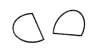Rotation,

Explanation:
Fiqures are rotation as a rotation is a type of transformation which is a turn. A figure can be turned clockwise or counterclockwise on the coordinate plane. In both transformations the size and shape of the figure stays exactly the same. A rotation is a transformation that turns the figure in either a clockwise or counterclockwise direction.

Question 7.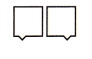Reflection,

Explanation:
Fiqures are reflection as a reflection is a mirror image of the shape. An image will reflect through a line, known as the line of reflection. A figure is said to reflect the other figure, and then every point in a figure is equidistant from each corresponding point in another figure.

Question 8.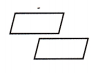Translation,

Explanation:
Both the figures are translated of one other because one is a translation of other that is identical duplicate of the other.

Identify lines of symmetry.

Question 9.How many lines of symmetry does the figure above have?
4 or four,

Explanation:
A line of symmetry is the line that divides the shape into two halves that match exactly. A square has four lines of linear symmetry. One crosses through each diagonal. The other is the two lines that cross horizontally and vertically through the middle of the square therefore a square has 4 lines of symmetry.

Question 10.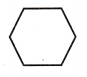Draw the lines of symmetry on the figure above.